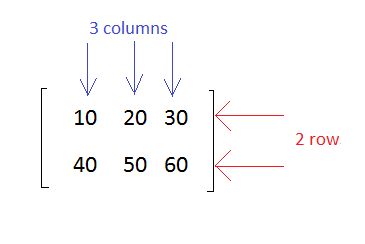# Matlab-Matrix - Introduction

MATLAB (matrix laboratory) is a fourth-generation high-level programming language and interactive environment for numerical computation, visualization and programming. It allows matrix manipulations; plotting of functions and data; implementation of algorithms; creation of user interfaces; interfacing with programs written in other languages, including C, C++, Java, and FORTRAN; analyze data; develop algorithms; and create models and applications.

In this tutorial we will focus on Matrix Implementation using MATLAB.

## Matrix

A matrix is a collection of numbers arranged in rows and columns that represents a rectangular array.

An example of matrix with 2 rows and 3 columns is as shown below## Matrix Dimension

The dimension of a matrix is defined based on the number of rows and columns.

A matrix with 2 rows and 3 columns is said to be 2x3 matrix.

A matrix with 3 rows and 3 columns is said to be 3x3 matrix.

## Matrix in Matlab

In MATLAB, you create a matrix by entering elements in each row as comma or space delimited numbers and using semicolons to mark the end of each row.

### Example

To create a 4x5 matrix, enter the following.

```a = [ 1 2 3 4 5; 2 3 4 5 6; 3 4 5 6 7; 4 5 6 7 8]
```

The matrix has 4 rows and 5 columns.

The first row will have values as 1 2 3 4 5

The second row: 2 3 4 5 6

The third row: 3 4 5 6 7

The fourth row: 4 5 6 7 8

### Output

The matrix of size 4x5 will look as follows

```a =
1  2  3  4  5
2  3  4  5  6
3  4  5  6  7
4  5  6  7  8
```

Let us test the matrix creation in MATLAB command window as shown below −

```>> a = [ 1 2 3 4 5; 2 3 4 5 6; 3 4 5 6 7; 4 5 6 7 8]

a =
1  2  3  4  5
2  3  4  5  6
3  4  5  6  7
4  5  6  7  8

>>
```

## Referencing the Elements

To reference an element in the mth row and nth column, of a matrix mx, we write the following

```mx(m, n);
```

## Example

To refer to the element in the 2nd row and 5th column, of the matrix a, as created in the last section, we type the following.

```>> a = [ 1 2 3 4 5; 2 3 4 5 6; 3 4 5 6 7; 4 5 6 7 8]

a =
1  2  3  4  5
2  3  4  5  6
3  4  5  6  7
4  5  6  7  8

>> a(2,5)

ans =
6

>>
```

To get all the elements of the nth column in a matrix , you can make use of A (:,n) where n represents the column no in the matrix.

```A(:,n).
```

### Example

Now, let us create a column vector v, from all the elements of the 4th column of the matrix a. This will be as follows

```a = [ 1 2 3 4 5; 2 3 4 5 6; 3 4 5 6 7; 4 5 6 7 8];
v = a(:,4)
```

### Output

MATLAB will execute the above statement and return the following result.

```>> a = [ 1 2 3 4 5; 2 3 4 5 6; 3 4 5 6 7; 4 5 6 7 8]

a =
1  2  3  4  5
2  3  4  5  6
3  4  5  6  7
4  5  6  7  8

>> v=a(:,4)

v =
4
5
6
7

>>
```

You can also select the elements in the mth through nth columns. For this, we write as follows.

```a(:,m:n)
```

### Example

Let us create a smaller matrix by taking the elements from the second and third columns, as shown below −

```a = [ 1 2 3 4 5; 2 3 4 5 6; 3 4 5 6 7; 4 5 6 7 8];
a(:, 2:3)
```

### Output

MATLAB will execute the above statement and return the following result −

```>> a = [ 1 2 3 4 5; 2 3 4 5 6; 3 4 5 6 7; 4 5 6 7 8]

a =
1  2  3  4  5
2  3  4  5  6
3  4  5  6  7
4  5  6  7  8

>> a(:, 2:3)

ans =
2  3
3  4
4  5
5  6

>>
```

In the same way, you can create a sub-matrix by taking a sub-part of a matrix.

## Example

Let us create a sub-matrix saby taking the inner subpart of a, as given below −

```3  4  5
4  5  6
```

During execution in MATLAB command window, the matrix will be as shown below −

```>> a = [ 1 2 3 4 5; 2 3 4 5 6; 3 4 5 6 7; 4 5 6 7 8]

a =
1  2  3  4  5
2  3  4  5  6
3  4  5  6  7
4  5  6  7  8

>> sa = a(2:3,2:4)

sa =
3  4  5
4  5  6

>>
```